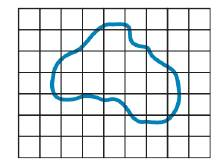Chapter 8.1, Problem 55EElementary Geometry For College St...

7th Edition
Alexander + 2 others
ISBN: 9781337614085

Solutions

Chapter
SectionElementary Geometry For College St...

7th Edition
Alexander + 2 others
ISBN: 9781337614085
Textbook Problem

a) Find a lower estimate of the area of the figure by counting whole squares within the figure.b) Find an upper estimate of the area of the figure by counting whole and partial squares within the figure.c) Use the average of the results in parts (a) and (b) to provide a better estimate of the area of the figure.d) Does intuition suggest that the area estimate of part (c) is the exact answer?To determine

a.

To find:

A lower estimate of the area of the given figure.

Explanation

Converting theoretical statement into mathematical statement and the calculation is followed by suitable geometric formulae.

Calculation:

Given,

Lower estimated area of the figure(A)=number of whole squares within the figure

To determine

b.

To find:

An upper estimate of the area of the given figure.

To determine

c.

A better estimated area of the given figure by the average of the results in part (a) and part (b).

To determine

d.

To suggest:

The area estimated in part (c) is the exact answer.

Still sussing out bartleby?

Check out a sample textbook solution.

See a sample solution

The Solution to Your Study Problems

Bartleby provides explanations to thousands of textbook problems written by our experts, many with advanced degrees!

Get Started

Find more solutions based on key concepts